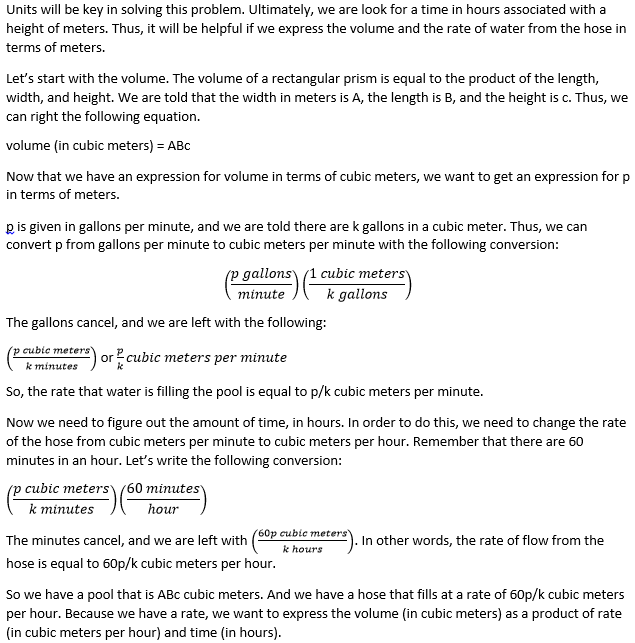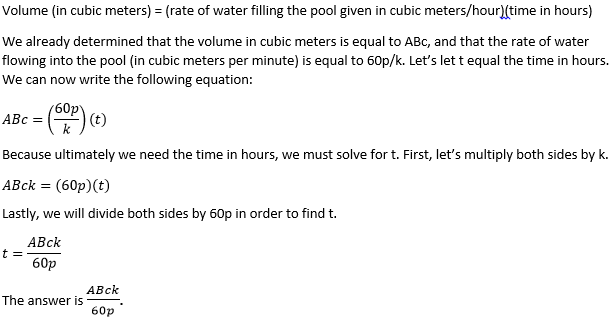# PSAT Math : How to find the volume of a prism

## Example Questions

### Example Question #1 : Volume Of A Rectangular Solid

A rectangular box has a length of 2 meters, a width of 0.5 meters, and a height of 3.2 meters. How many cubes with a volume of one cubic centimeter could fit into this rectangular box?

3.2 x 10-3

3.2

3,2 x 103

3.2 x 106

3.2 x 102

3.2 x 106

Explanation:

In order to figure out how many cubic centimeters can fit into the box, we need to figure out the volume of the box in terms of cubic centimeters. However, the measurements of the box are given in meters. Therefore, we need to convert these measurements to centimeters and then determine the volume of the box.

There are 100 centimeters in one meter. This means that in order to convert from meters to centimeters, we must multiply by 100.

The length of the box is 2 meters, which is equal to 2 x 100, or 200, centimeters.

The width of the box is 0.5(100) = 50 centimeters.

The height of the box is 3.2(100) = 320 centimeters.

Now that all of our measurements are in centimeters, we can calculate the volume of the box in cubic centimeters. Remember that the volume of a rectangular box (or prism) is equal to the product of the length, width, and height.

V = length x width x height

V = (200 cm)(50 cm)(320 cm) = 3,200,000 cm3

To rewrite this in scientific notation, we must move the decimal six places to the left.

V = 3.2 x 106 cm3

The answer is 3.2 x 106.

### Example Question #1949 : Hspt Mathematics

A rectangular prism has a length that is twice as long as its width, and a width that is twice as long as its height. If the surface area of the prism is 252 square units, what is the volume, in cubic units, of the prism?

216

27

108

1728

432

216

Explanation:

Let l be the length, w be the width, and h be the height of the prism. We are told that the length is twice the width, and that the width is twice the height. We can set up the following two equations:

l = 2w

w = 2h

Next, we are told that the surface area is equal to 252 square units. Using the formula for the surface area of the rectangular prism, we can write the following equation:

surface area = 2lw + 2lh + 2wh = 252

We now have three equations and three unknowns. In order to solve for one of the variables, let's try to write w and l in terms of h. We know that w = 2h. Because l = 2w, we can write l as follows:

l = 2w = 2(2h) = 4h

Now, let's substitute w = 2h and l = 4h into the equation we wrote for surface area.

2(4h)(2h) + 2(4h)(h) + 2(2h)(h) = 252

Simplify each term.

16h2 + 8h2 + 4h2 = 252

Combine h2 terms.

28h2 = 252

Divide both sides by 28.

h2 = 9

Take the square root of both sides.

h = 3.

This means that h = 3. Because w = 2h, the width must be 6. And because l = 2w, the length must be 12.

Because we now know the length, width, and height, we can find the volume of the prism, which is what the question ultimately requires us to find.

volume of a prism = l • w • h

volume = 12(6)(3)

= 216 cubic units

### Example Question #1950 : Hspt Mathematics

The dimensions of Treasure Chest A are 39” x 18”. The dimensions of Treasure Chest B are  16” x 45”. Both are 11” high. Which of the following statements is correct?

Treasure Chest B can hold more treasure.

There is insufficient data to make a comparison between Treasure Chest A and Treasure Chest B.

Treasure Chest A and B can hold the same amount of treasure.

Treasure Chest A has the same surface area as Treasure Chest B.

Treasure Chest A can hold more treasure.

Treasure Chest B can hold more treasure.

Explanation:

The volume of B is 7920 in3. The volume of A is 7722 in3. Treasure Chest B can hold more treasure.

### Example Question #772 : Geometry

Carlos has a pool in the shape of a rectangular prism. He fills the pool with a hose that ejects water at a rate of p gallons per minute. The bottom of the pool is A meters wide and B meters long. If there are k gallons in a cubic meter, then which of the following expressions will be equal to the amount of time it takes, in hours, for the water in the pool to reach a height of c meters?

60ABcp/k

ABck/(60p)

60ABc/(pk)

ABcp/(60k)

ABc/(pk)